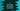# C program to find the power of a number using loop### C program to find the power of a number :

In this ‘C’ example program , we will learn how to find the power of a number . We will take both inputs from the user. Then we will calculate the power and print out the result. Let’s take a look into the program first :

### C program :

``````#include

int main(){
//1
int power,number;
int result=1;
int i = 0;

//2
printf("Enter a number : ");
scanf("%d",&number);
printf("Power : ");
scanf("%d",&power);

//3
for(i = 0;i<power;i++){
result = result * number;
}

//4
printf("%d to the power of %d is = %d\n",number,power,result);

return 0;
}``````

### Explanation :

The commented numbers above denotes the steps below :

1. Create variable power to store the power value , number to store the value of the number, result to store the final result (we have initialized it as 1) and one integer i to use in the loop below.
2. Get the value of number and power from the user and store it in power and number variables.
3. Run one for loop . This loop will run for ‘power’ times. Each time, multiply the value of result with the value of number, and set it to result.

For example, let’s say value of power is 3 and value of number is 4. Now the loop will run for 3 times. First time, the value of result will be result * number = 1 * 4 = 4. Second time, it will be result * number = 4 * 4 = 16 and third time, it is 16 * 4 = 64. So, it will print 64.

### Example inputs :

``````Enter a number : 4
Power : 3
4 to the power of 3 is = 64

Enter a number : 5
Power : 3
5 to the power of 3 is = 125

Enter a number : 3
Power : 3
3 to the power of 3 is = 27

Enter a number : 1
Power : 100
1 to the power of 100 is = 1``````